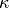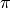# Quickstart¶

This page gives a good introduction in how to get started with SegEval. This assumes you already have SegEval installed. If you do not, head over to the Installation section.

Let’s get started with some simple examples.

Start by encoding the size of each segment produced by each coder for a document (e.g. the Stargazer’s text segmented in [Hearst1997]) as JSON using the format shown below.

{
"items": {
"stargazer": {
"1": [2,3,3,1,3,6,3],
"2": [2,8,2,4,2,3],
"3": [2,1,2,3,1,3,1,3,2,2,1],
"4": [2,1,4,1,1,3,1,4,3,1],
"5": [3,2,4,3,5,4],
"6": [2,3,4,2,2,5,3],
"7": [2,3,2,2,3,1,3,2,3]
}
},
"segmentation_type": "linear"
}


Begin by importing the SegEval module:

>>> import segeval


Now, let’s import this data using the input_linear_mass_json() function:

>>> dataset = segeval.input_linear_mass_json('hearst1997.json')


Now, we have a Dataset object called dataset. We can compute a variety of statistics upon this data.

## Comparing Segmentations¶

Given a dataset, if we wanted to compare two coder’s responses together, we can select the coder’s that we care about much like how one accesses arrays/dictionary items:

>>> import segeval
>>> dataset = segeval.HEARST_1997_STARGAZER
>>> segmentation1 = dataset['stargazer']['1']
>>> segmentation2 = dataset['stargazer']['2']


Segmentations can then be compared using functions such as:

>>> segeval.boundary_similarity(segmentation1, segmentation2)
Decimal('0.5')


Other metrics are also available, including:

>>> segeval.segmentation_similarity(segmentation1, segmentation2)
Decimal('0.825')


If instead of one metric you desire a large number of statistics about the difference between two boundaries, you can use:

>>> segeval.boundary_statistics(segmentation1, segmentation2)


This produces:

{
'matches': [1, 1, 1], # List of matching boundary types
'boundaries_all': 11,
'pbs': 20, # Potential boundaries
'transpositions': [Transposition(start=8, end=9, type=1)],
'full_misses': [1, 1, 1, 1, 1], # List of full miss boundary types
'count_edits': Decimal('3.5'), # Scaled count of all edits
'substitutions': []
}


If instead we had a hypothetical segmentation generated by an automatic segmenter and we wanted to compare it against segmentation1, we could use these metrics:

>>> hypothesis = (2,6,4,2,4,3)
>>> reference = dataset['stargazer']['1']
>>> segeval.boundary_similarity(hypothesis, reference)
Decimal('0.5714285714285714285714285714')


Some traditional segmentation comparison metrics can also be used:

>>> segeval.window_diff(hypothesis, reference)
Decimal('0.3157894736842105263157894737')
>>> segeval.pk(hypothesis, reference)
Decimal('0.2631578947368421052631578947')


If instead one wants to analyze this as a boundary classification task, we can produce a confusion matrix using:

>>> confusion_matrix = segeval.boundary_confusion_matrix(hypothesis, reference)


This produces a ConfusionMatrix object named confusion_matrix. This confusion matrix can then be passed to information retrieval metrics, such as:

>>> segeval.precision(confusion_matrix)
Decimal('0.5714285714285714285714285714')
>>> segeval.recall(confusion_matrix)
Decimal('0.5714285714285714285714285714')
>>> segeval.fmeasure(confusion_matrix)
Decimal('0.7272727272727272727272727267')


All of these functions can be used on either pairs of segmentations, single Dataset objects (computing pairwise values), and two Dataset objects (comparing the coders in one to all coders in another). Comparing two Dataset objects is how one could compare a set of automatic segmenters to a set of human segmenters to evaluate the performance of the automatic segmenters, for example:

>>> manual = segeval.HEARST_1997_STARGAZER
>>> automatic = segeval.HYPOTHESIS_STARGAZER
>>> segeval.boundary_similarity(manual, automatic)


This produces:

{
'stargazer,3,h2': Decimal('0.5'),
'stargazer,3,h1': Decimal('0.45'),
'stargazer,6,h1': Decimal('0.5833333333333333333333333333'),
'stargazer,1,h1': Decimal('0.5714285714285714285714285714'),
'stargazer,1,h2': Decimal('0.3888888888888888888888888889'),
'stargazer,6,h2': Decimal('0.3888888888888888888888888889'),
'stargazer,7,h2': Decimal('0.3181818181818181818181818182'),
'stargazer,7,h1': Decimal('0.5'),
'stargazer,5,h1': Decimal('0.4166666666666666666666666667'),
'stargazer,5,h2': Decimal('0.375'),
'stargazer,2,h1': Decimal('0.4285714285714285714285714286'),
'stargazer,2,h2': Decimal('0.3333333333333333333333333333'),
'stargazer,4,h2': Decimal('0.3636363636363636363636363636'),
'stargazer,4,h1': Decimal('0.4444444444444444444444444444')
}


Note that the key for each value is the document name (stargazer), followed by the coder from the manual dataset (e.g., 3) and the coder from the automatic dataset (e.g., h2).

## Computing Inter-Coder Agreement¶

Given a dataset, if we wanted to compute the actual agreement between all coders using boundary_similarity() we can use:

>>> import segeval
>>> dataset = segeval.HEARST_1997_STARGAZER
>>> segeval.actual_agreement_linear(dataset)
Decimal('0.5300546448087431693989071038')


If instead one would like to use segmentation_similarity(), we can specify this function:

>>> segeval.actual_agreement_linear(dataset, fnc_compare=segeval.segmentation_similarity)
Decimal('0.7952380952380952380952380952')


If instead we want a chance-corrected inter-coder agreement coefficient, Fleiss’andadapted to use boundary_similarity() can be used:

>>> segeval.fleiss_kappa_linear(dataset)
Decimal('0.4414910889068254984367317023')
>>> segeval.fleiss_pi_linear(dataset)
Decimal('0.4405412438199323445225084569')